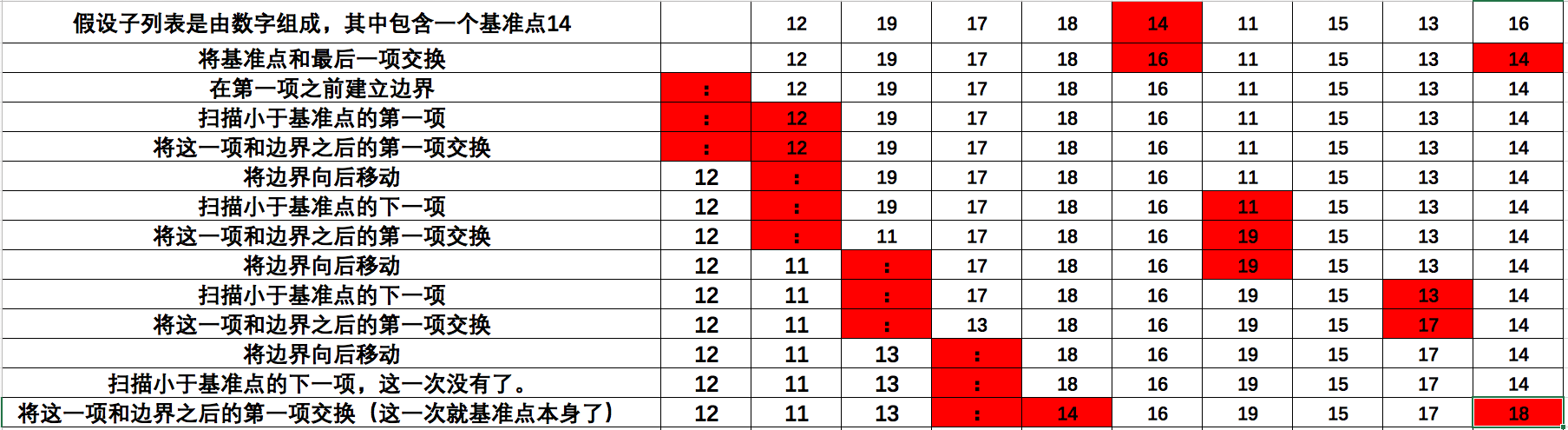### 快速排序

1. 首先，从列表的中点位置选取一项。这一项叫做基准点(pivot)

2. 将列表中的项分区，以便小鱼基准点的所有项都移动到基准点的左边，而剩下的移动到基准点的右边。更具相关的实际项，基准点自身的位置也是变化的。例如：如果基准点自身是最大的项，它会位于列表的最右边，如果基准点是最小值，它会位于最左边。但是不管基准点最终位于何处。这个位置都是它在完全排序的列表中的最终位置。

3. 分而治之。对于在基准点分割列表而形成的子列表，递归地重复调用该过程。一个子列表包含了基准点左边的所有项，另一个子列表包含基准点右边的所有项。

4. 每次遇到少于 2 个项的一个子列表，就结束这个过程。

#### 分割

1. 将基准点和子列表中的最后一项交换。

2. 在已知小于基准点的项和剩余的项之间建立一个边界。在开始的时候边界放在最左边的首位（就是在第一项之前）

3. 从子列表中的第一项开始，历遍整个子列表。每次遇到小于基准点的项，就将其与边界之后的第一项交换，并将边界移动到交换项之后。

4. 将基准点和边界之后的第一项交换，从而完成整个过程。### Python实现：

def quick_sort(lyst):
quick_sort_helper(lyst, 0, len(lyst) - 1)

def partition(lyst, left, right):
"""
找到基准点并与项目中最后一个交换
"""

def swap(lyst, i, j):
"""交换项目中 i 和 j的位置"""
#  可以理解为 lyst[i], lyst[j] = lyst[j], lyst[i]
temp = lyst[i]
lyst[i] = lyst[j]
lyst[j] = temp
#   找到基准点并与最后一项交换
middle = (left + right) // 2
pivot = lyst[middle]
lyst[middle], lyst[right] = lyst[right], lyst[middle]
#  将边界点设置为第一个位置
boundary = left
#  将项移动到基准点左侧
for index in range(left, right):
if lyst[index] < pivot:
swap(lyst, index, boundary)
boundary += 1
#  交换基准点和边界项目
swap(lyst, right, boundary)
return boundary

def quick_sort_helper(lyst, left, right):
"""用于隐藏子列表重点的额外参数
:param lyst:
:param left:
:param right:
:return:
"""
if left < right:
pivot_location = partition(lyst, left, right)
quick_sort_helper(lyst, left, pivot_location - 1)
quick_sort_helper(lyst, pivot_location + 1, right)

def quick_sort_main(size=20, sort=quick_sort):
import random
lyst = []
for count in range(size):
lyst.append(random.randint(1, size + 1))
print(lyst)
print(f"排序前lyst内存地址：{id(lyst)}\n")
sort(lyst)
print(lyst)
print(f"排序后lyst内存地址：{id(lyst)}\n")

if __name__ == '__main__':
quick_sort_main()



Go实现：

package main

import (
"math/rand"
"time"
"fmt"
)

func partition(lyst []int, left int, right int) int {

swap := func(lyst []int, i int, j int) {

temp := lyst[i]
lyst[i] = lyst[j]
lyst[j] = temp
}
middle := (left + right) / 2
pivot := lyst[middle]
lyst[middle], lyst[right] = lyst[right], lyst[middle]
boundary := left
for i := left; i < right; i ++{
if lyst[i] < pivot {
swap(lyst, i, boundary)
boundary += 1
}
}
swap(lyst, right, boundary)
return boundary
}

func quickSortHelper(lyst []int, left int, right int) {
if left < right {
pivotLocation := partition(lyst, left, right)
quickSortHelper(lyst, left, pivotLocation-1)
quickSortHelper(lyst, pivotLocation+1, right)
}
}

func quickSort(lyst []int) {
quickSortHelper(lyst, 0, len(lyst)-1)
}

func main() {
//生成若干个不重复的随机数
//生成count个[start,end)结束的不重复的随机数
generateRandomNumber := func(start int, end int, count int) []int {
//范围检查
if end < start || (end-start) < count {
return nil
}

//存放结果的slice
nums := make([]int, 0)
//随机数生成器，加入时间戳保证每次生成的随机数不一样
r := rand.New(rand.NewSource(time.Now().UnixNano()))
for len(nums) < count {
//生成随机数
num := r.Intn((end - start)) + start

//查重
exist := false
for _, v := range nums {
if v == num {
exist = true
break
}
}

if !exist {
nums = append(nums, num)
}
}

return nums
}

nums := generateRandomNumber(1, 100, 40)
fmt.Println("排序前：",nums)
quickSort(nums)
fmt.Println("排序后：",nums)
}



1. 性能：

1. 内存：